# 0.PTA得分情况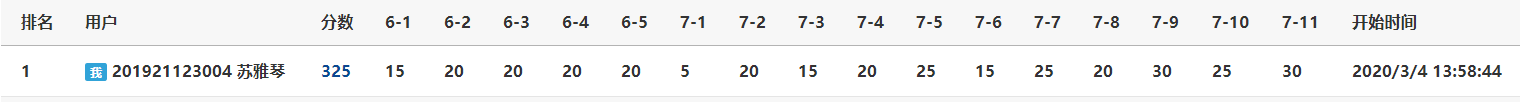# 1.本周学习总结

## 栈->只允许在一端进行操作的结构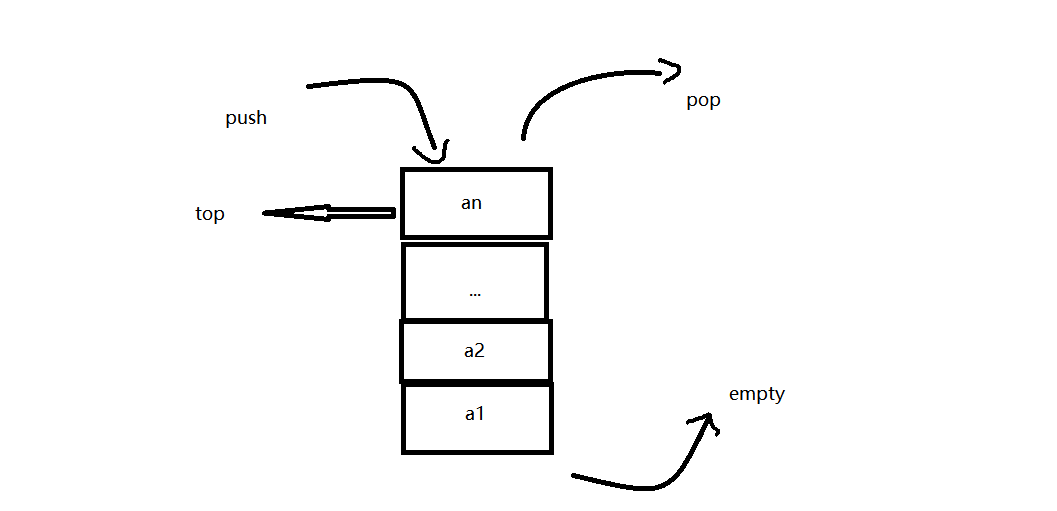### 栈的存储结构

typedef int Position;
typedef struct SNode *PtrToSNode;
struct SNode {
ElementType *Data;  /* 存储元素的数组 */
Position Top;       /* 栈顶指针       */
int MaxSize;        /* 堆栈最大容量   */
};
typedef PtrToSNode Stack;


### 栈的基本操作

#### 初始化栈

Stack InitStack(int MaxSize)
{
Stack s = (Stack)malloc(sizeof(struct SNode));
s->Data = (int*)malloc(sizeof(int) * MaxSize);
s->MaxSize = MaxSize;
s->Top = 00;
return s;
}


#### 进栈

bool Push(Stack S, ElementType X)
{

if (S->Top == S->MaxSize )
{
printf("Stack Full\n");
return false;
}
S->Top++;  //要注意顺序
S->Data[S->Top] = X;
return true;

}


#### 出栈

ElementType Pop(Stack S)
{

int e;
if (S->Top == -1)//对于top为多少时栈空，要具体看题意
{
printf("Stack Empty\n");
return ERROR;
}
e = S->Data[S->Top];
S->Top--;  //若只是读取栈顶，这句不要
return e;
}


#### 判空和销毁栈

bool StackEmpty(Stack S)
{
return S->top==-1;
}
void DesStack(SqStack &S)
{
free(s);
}


### 1.判断字符串是否是对称串

• 判断对称的方法，可以进行首位对比，那么就可以利用栈的特点，后进先出，将后面的元素与开始的元素进行对比
bool symmetry(ElemType str[])
{
InitStack(s);  //初始化栈

for (int i = 0; str[i] != '\0'; i++)
{
Push(s, str[i]);  //将元素入栈
}
for (int i = 0; str[i] != '\0'; i++)
{
Pop(s, e);
if (str[i] != e)   //反着对比是否一样
{
DesStack(s);  //销毁栈
};
return false;
}
DesStack(s);  //销毁栈
return true;
}


### 2.符号匹配

• 符号匹配的大体思路是如果左括号进栈，遇到右括号，则与栈顶元素进行比较，如果匹配，则出栈，不匹配，则输出no。
#include<iostream>
using namespace std;
#include<stack>
#include<string>
#include<algorithm>
int main()
{
stack<char>s;
string str;
int len;
char ch;
int flag = 0;
pair<char, char>p1('[', ']');
pair<char, char>p2('{', '}');
pair<char, char>p3('(', ')');
cin >> str;
len = str.size();

for (int i = 0; i < len; i++)
{
if (str[i] == '{' || str[i] == '(' || str[i] == '[')
{
s.push(str[i]);  //如果是左括号，则进队
flag = 1;
}
else if (str[i] == p1.second )  //当遇到右括时，进行判断
{
if (!s.empty()&&s.top() == p1.first)
{
s.pop();  //如果栈不空并且与左括号匹配，则出栈
}
else if(s.empty()) //如果栈是空的则不匹配
{

cout << "no";
return 0;
}

}
else if ( str[i] == p2.second) //下面与上面相似
{
if (!s.empty() &&s.top() == p2.first)
{
s.pop();
}
else if(s.empty())
{

cout << "no";
return 0;
}
}
else if ( str[i] == p3.second)
{
if (!s.empty() &&s.top() == p3.first)
{
s.pop();
}
else if (s.empty())
{
cout << "no";
return 0;
}
}
}

if (flag && s.empty())
{
cout << "yes";
return 0;

}
else
{
cout << s.top() << endl;
cout << "no";
}
return 0;
}

• 这个是在一开始在pta里写的题目，其实那时候不太会用pair容器，这样写，其实显得有点多余，如果用map容器可能会好一点，并且对于判断右括号与栈顶元素是否匹配的部分，可以用函数封装，代码会更加简洁。

### 3.符号匹配/*

#include<iostream>
using namespace std;
#include<stack>
#include<map>
int flag = 1;
bool Ismatch(stack<char>& s, map<char, char>& m, char str)  //查看是否匹配,处理右括号的三种情况
{
if (!s.empty() && m[s.top()] == str) //  输入样例  （）
{
flag = 1;
s.pop();
return true;
}
else if (s.empty())  //  输入样例  ()]
{
flag = 0;
cout << "NO" << endl;
if (str == '#')
{
cout << "?-*/";
}
else
cout << "?-" << str;
return false;
}
else if (!s.empty())    输入样例(])
{
flag = 0;
cout << "NO" << endl;
if (s.top() == '#')
{
cout << "/*-?";
}
else
cout << s.top() << "-?";
return false;
}
}
int main()
{
map<char, char>m;
stack<char>s;
char str;
int len;
char ch;
int i = 0;
m['('] = ')';
m['{'] = '}';
m['['] = ']';
m['#'] = '#';
while (cin >> ch)
{
str[i] = ch;
i++;
}
len = i;
i = 0;
while (i < len)
{
if (str[i] == '{' || str[i] == '(' || str[i] == '[')
{
s.push(str[i]);
flag = 1;
}
else if (str[i] == m['{'])
{
if (!Ismatch(s, m, str[i]))
return 0;
}
else if (str[i] == m['('])
{
if (!Ismatch(s, m, str[i]))
return 0;
}
else if (str[i] == m['['])
{
if (!Ismatch(s, m, str[i]))
return 0;
}
else if (str[i] == '/' && str[i + 1] == '*')
{
str[i] = str[i + 1] = '#';   //将两个字符换成一个字符
s.push('#');
flag = 1;
i++;       //要加一
}
else if (str[i] == '*' && str[i + 1] == '/')
{
str[i] = str[i + 1] = '#';
i++;
if (!Ismatch(s, m, str[i]))
return 0;
}
i++;
}
if (flag && s.empty())
cout << "YES";
else if (flag || !s.empty())  //处理开头多余的左括号，或结尾多余的左括号
{
cout << "NO\n";
if (s.top() == '#')
{
cout << "/*-?";
}
else
cout << s.top() << "-?";
}
return 0;
}

• 这道题，其实大致的思路和上面一道题是一样的，不过对于 /* 的处理，有点不一样，这里，我将 /* 这个全部换成一个字符#，并且只让一个#进栈，而不是两个#，同时，这道题，更难得是，左括号和右括号都是要处理，可能左括号不匹配，可能右括号不匹配，如果有左括号，没右括号，那么此时的左括号就会在栈里，那么处理的时间就是在最后，如果有右括号没左括号，那么就要判断三种情况，栈顶左括号与右括号匹配，则出栈，空队列的情况下输出右括号，栈顶左括号与右括号不匹配。

### 4.迷宫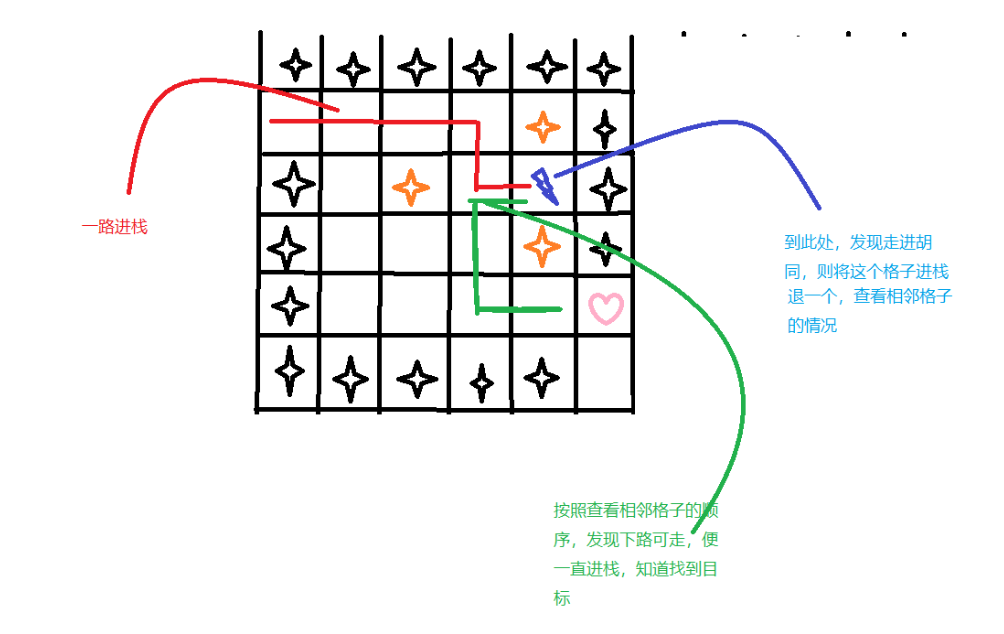#include <stdio.h>
#define MaxSize 100
#define M 8
#define N 8
int mg[M+2][N+2]=
{
{1,1,1,1,1,1,1,1,1,1},
{1,0,0,1,0,0,0,1,0,1},
{1,0,0,1,0,0,0,1,0,1},
{1,0,0,0,0,1,1,0,0,1},
{1,0,1,1,1,0,0,0,0,1},
{1,0,0,0,1,0,0,0,0,1},
{1,0,1,0,0,0,1,0,0,1},
{1,0,1,1,1,0,1,1,0,1},
{1,1,0,0,0,0,0,0,0,1},
{1,1,1,1,1,1,1,1,1,1}
};
typedef struct
{
int i;				//当前方块的行号
int j;				//当前方块的列号
int di;				//di是下一可走相邻方位的方位号
} Box;
typedef struct
{
Box data[MaxSize];
int top;			//栈顶指针
} StType;				//定义栈类型
int mgpath(int xi,int yi,int xe,int ye)	//求解路径为:(xi,yi)->(xe,ye)
{
int i,j,k,di,find;
StType st;					//定义栈st
st.top=-1;					//初始化栈顶指针
st.top++;      				//初始方块进栈
st.data[st.top].i=xi; st.data[st.top].j=yi;	st.data[st.top].di=-1;
mg[xi][yi]=-1;
while (st.top>-1)			//栈不空时循环
{
i=st.data[st.top].i;j=st.data[st.top].j;di=st.data[st.top].di;  //取栈顶方块
if (i==xe && j==ye)		//找到了出口,输出路径
{
printf("迷宫路径如下:\n");
for (k=0;k<=st.top;k++)
{
printf("\t(%d,%d)",st.data[k].i,st.data[k].j);
if ((k+1)%5==0)	//每输出每5个方块后换一行
printf("\n");
}
printf("\n");
//return(1);		//找到一条路径后返回1
}
find=0;
while (di<4 && find==0)		//找下一个可走方块
{
di++;
switch(di)
{
case 0:
i=st.data[st.top].i-1;
j=st.data[st.top].j;break;
case 1:
i=st.data[st.top].i;
j=st.data[st.top].j+1;break;
case 2:
i=st.data[st.top].i+1;
j=st.data[st.top].j;break;
case 3:
i=st.data[st.top].i；
j=st.data[st.top].j-1;break;
}
if (mg[i][j]==0) find=1;	//找到下一个可走相邻方块
}
if (find==1)					//找到了下一个可走方块
{
st.data[st.top].di=di;		//修改原栈顶元素的di值
st.top++;					//下一个可走方块进栈
st.data[st.top].i=i;
st.data[st.top].j=j;
st.data[st.top].di=-1;
mg[i][j]=-1;				//避免重复走到该方块
}
else							//没有路径可走,则退栈
{
mg[st.data[st.top].i][st.data[st.top].j]=0;//让该位置变为其他路径可走方块
st.top--;					//将该方块退栈
}
}
return(0);							//表示没有可走路径,返回0
}
int main()
{
mgpath(1,1,M,N);
return 0;
}



## 队列->允许在头尾进行操作的结构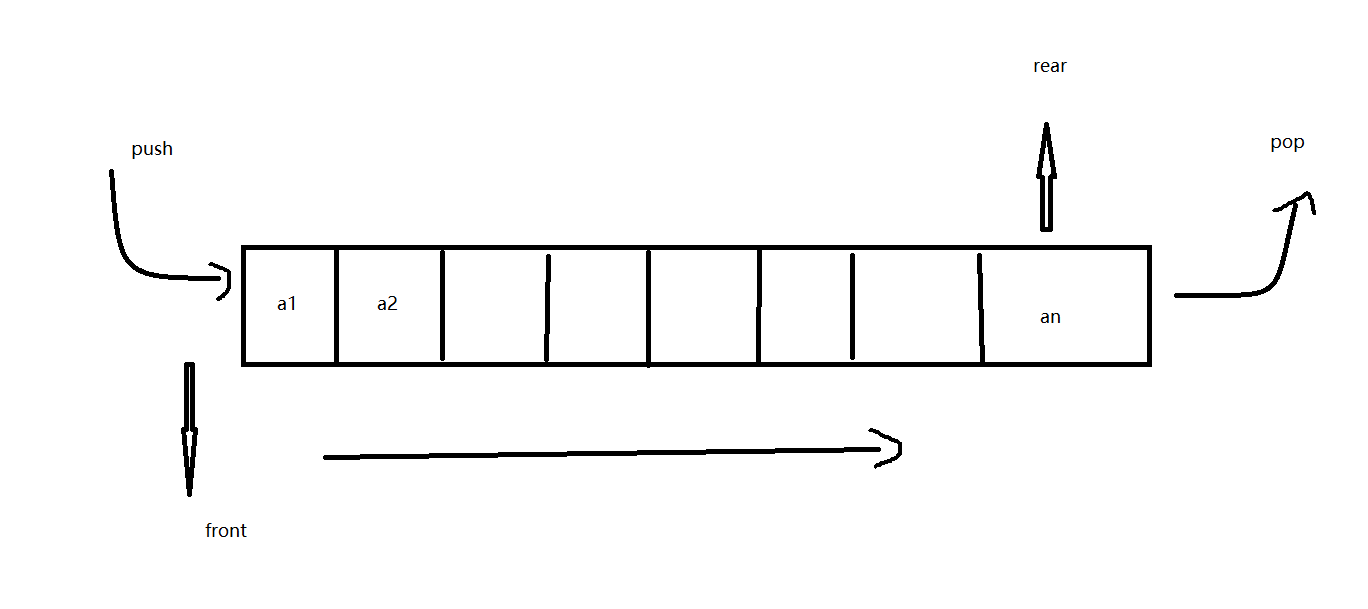### 队列的存储结构

typedef struct {
ElementType data[MAXQSIZE];
int front; //头指针
int rear; //尾指针
} Queue;


### 队列的基本操作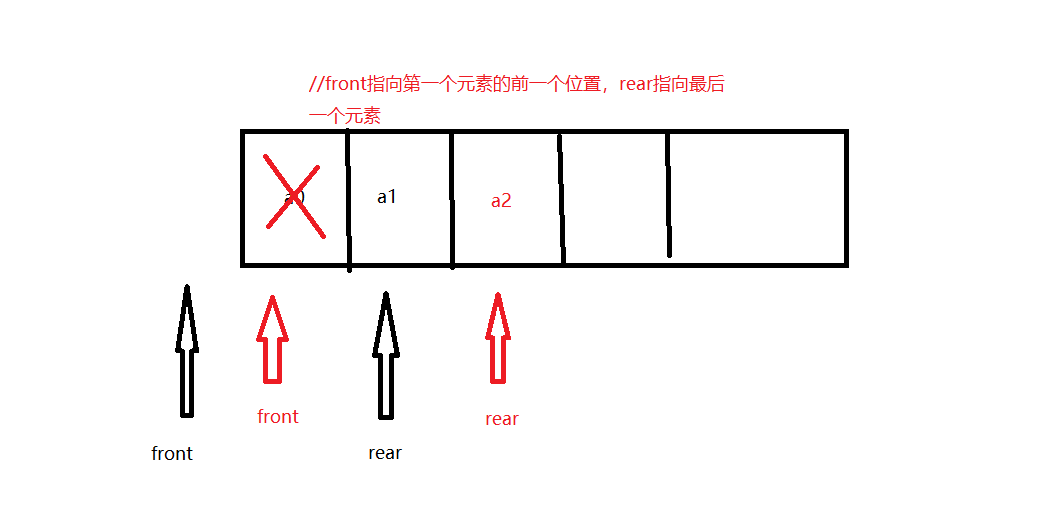#### 初始化队列

void InitQueue(SqQueue *&q)//  q是结构体指针
{
q=new Queue;
q->front=q->rear=-1;  //对于是不是-1，其实也不一定，要看题意
}


#### 进队

bool enQueue(SqQueue *&q,ElemType e)
{
if(q->rear==MaxSize-1)
return false;
q->rear++;   //注意顺序
q->data[q->rear]=e;
return true;
}


#### 出队

bool deQueue(SqQueue *&q,ElemType &e)
{
if(q->front==q->rear)
return false;
q->front++;
e=q->data[q->front];
return true;
}


#### 判空和销毁队列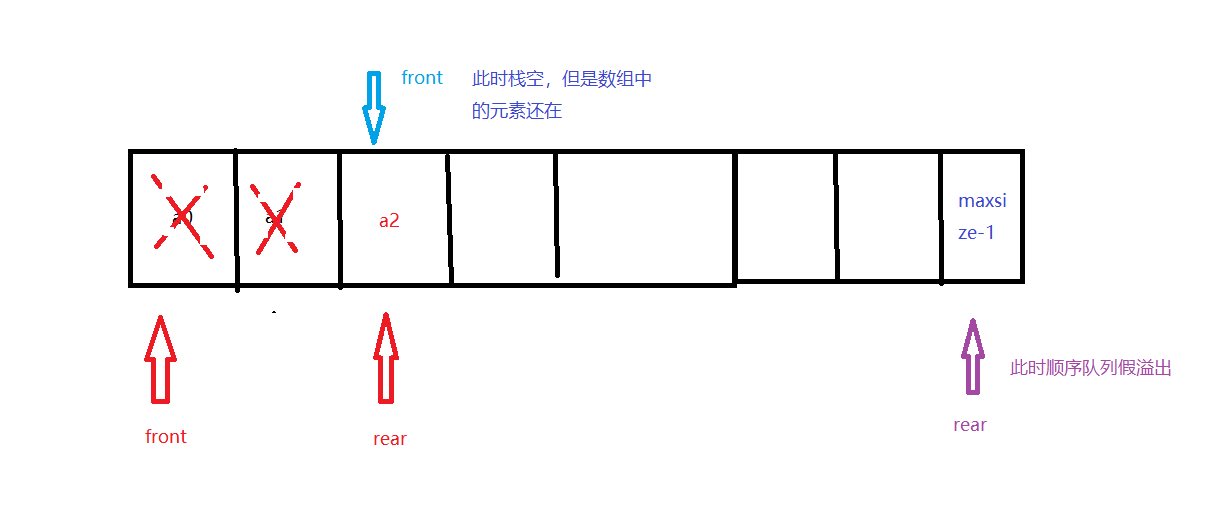bool QueueEmpty(SqQueue *q)
{
return  q->front==q->rear;
}
bool DestoryQueue(SqQueue *&q)
{
free(q);
}


### 循环队列

• 由于顺序队列会出现假溢出的现象，所以引进循环队列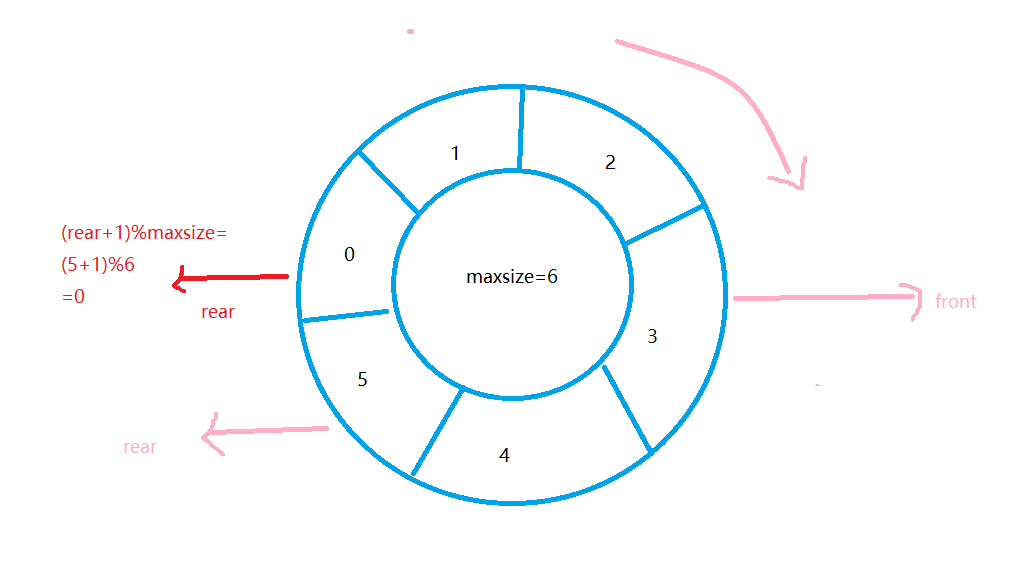#### 注意与一般队列的不同：

• 对空条件：front=rear；
• 队满条件：（rear+1)%MaxSize=front
• 进队条件：rear=(rear+1)%MaxSiaze;
• 出队条件：front=(front+1)%MaxSize;

### 1.报数游戏

• 11，2，1，2进行报数，数到1的人出队，从头开始，如此下去，直到所有的人都出队。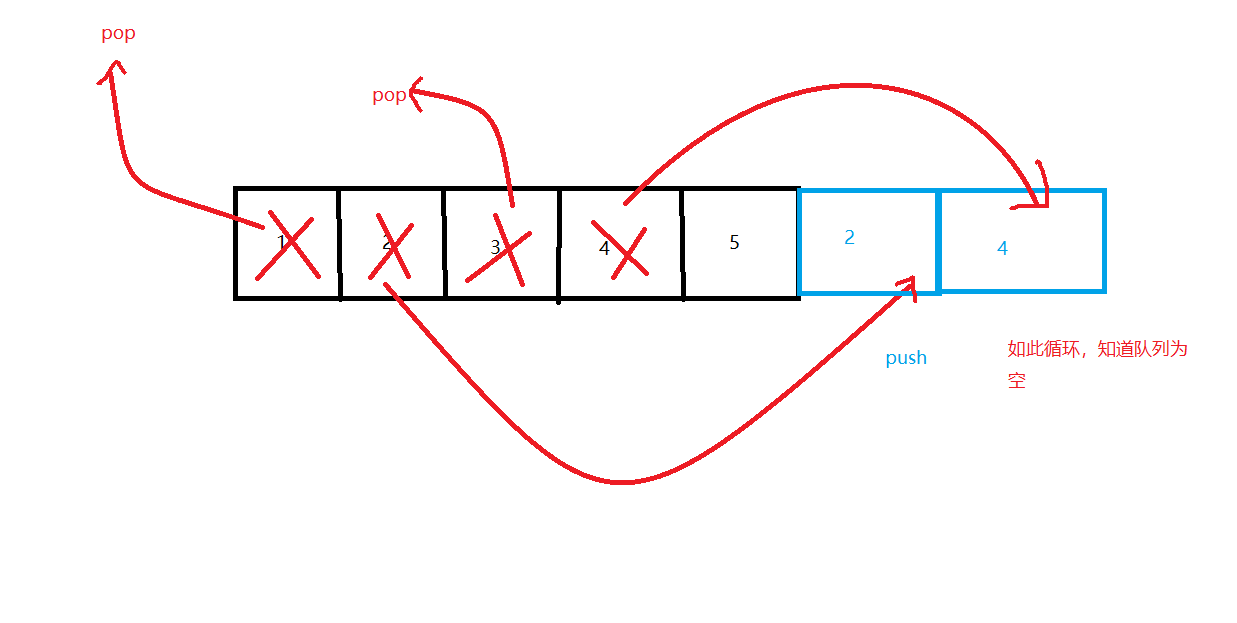这个是从1数到m的代码，范围更大写

#include<iostream>
using namespace std;
#include<queue>
int main()
{
queue<int>q;
int n;
int m;
int i ;
int top;
int flag = 1;
cin >> n >> m;
if (m > n)
{
cout << "error!";
return 0;
}
for (i = 1; i <= n; i++)
{
q.push(i);  //将元素进栈
}

while (q.size() >= m)
{
for (i = 1; i < m; i++)
{
top = q.front();  //将前m个元素都出队，再进队
q.pop();     //则位置变到后面
q.push(top);
}
if (flag)
{
cout << q.front();  //将栈顶元素输出
flag = 0;
}
else
{
cout << " " << q.front();
}
q.pop();  //将栈顶元素出队
}
while (!q.empty())//栈中可能不空，则要全部输出
{
cout <<" "<< q.front();
q.pop();
}
return 0;
}


### 2.迷宫问题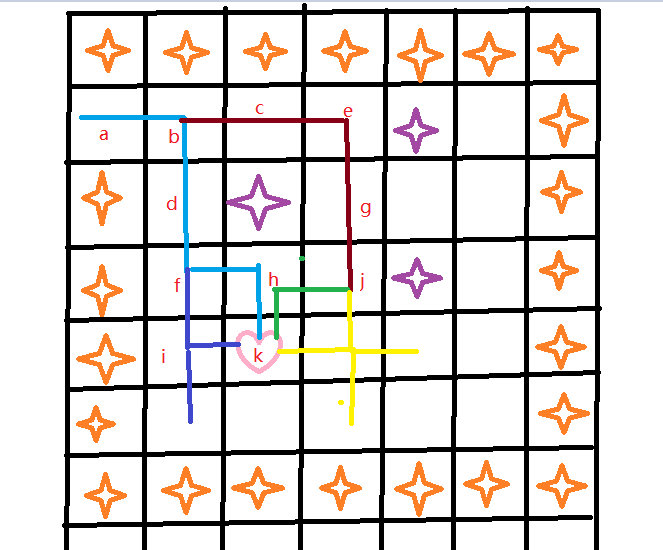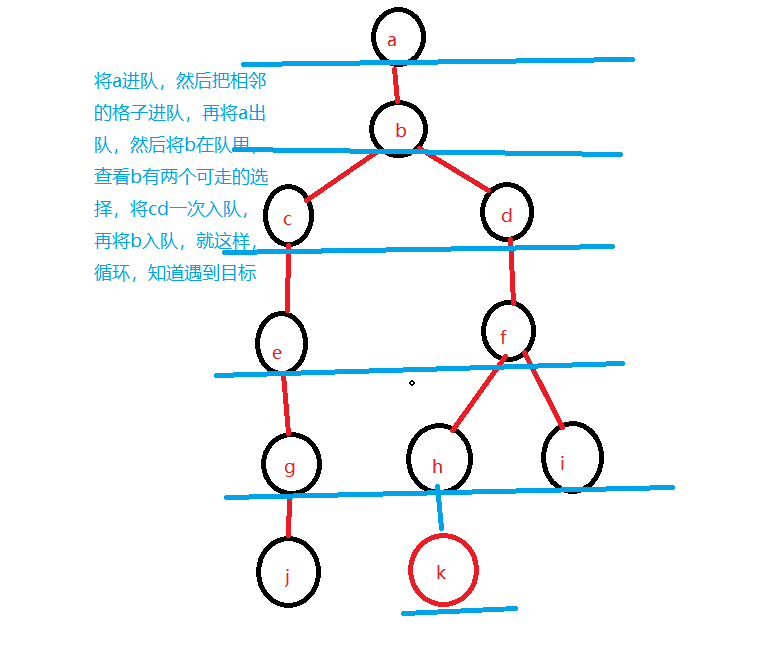#include <stdio.h>
#define MaxSize 100
#define M 8
#define N 8
int mg[M+2][N+2]=
{
{1,1,1,1,1,1,1,1,1,1},
{1,0,0,1,0,0,0,1,0,1},
{1,0,0,1,0,0,0,1,0,1},
{1,0,0,0,0,1,1,0,0,1},
{1,0,1,1,1,0,0,0,0,1},
{1,0,0,0,1,0,0,0,0,1},
{1,0,1,0,0,0,1,0,0,1},
{1,0,1,1,1,0,1,1,0,1},
{1,1,0,0,0,0,0,0,0,1},
{1,1,1,1,1,1,1,1,1,1}
};
typedef struct
{	int i,j;			//方块的位置
int pre;			//本路径中上一方块在队列中的下标
} Box;					//方块类型
typedef struct
{
Box data[MaxSize];
int front,rear;		//队头指针和队尾指针
} QuType;				//定义顺序队类型
void print(QuType qu,int front)	//从队列qu中输出路径
{
int k=front,j,ns=0;
printf("\n");
do				//反向找到最短路径,将该路径上的方块的pre成员设置成-1
{	j=k;
k=qu.data[k].pre;
qu.data[j].pre=-1;
} while (k!=0);
printf("迷宫路径如下:\n");
k=0;
while (k<MaxSize)  //正向搜索到pre为-1的方块,即构成正向的路径
{	if (qu.data[k].pre==-1)
{	ns++;
printf("\t(%d,%d)",qu.data[k].i,qu.data[k].j);
if (ns%5==0) printf("\n");	//每输出每5个方块后换一行
}
k++;
}
printf("\n");
}
int mgpath(int xi,int yi,int xe,int ye)					//搜索路径为:(xi,yi)->(xe,ye)
{
int i,j,find=0,di;
QuType qu;						//定义顺序队
qu.front=qu.rear=-1;
qu.rear++;
qu.data[qu.rear].i=xi;
qu.data[qu.rear].j=yi;	//(xi,yi)进队
qu.data[qu.rear].pre=-1;
mg[xi][yi]=-1;					//将其赋值-1,以避免回过来重复搜索
while (qu.front!=qu.rear && !find)	//队列不为空且未找到路径时循环
{
qu.front++;					//出队,由于不是环形队列，该出队元素仍在队列中
i=qu.data[qu.front].i;
j=qu.data[qu.front].j;
if (i==xe && j==ye)			//找到了出口,输出路径
{
find=1;
print(qu,qu.front);			//调用print函数输出路径
return(1);				//找到一条路径时返回1
}
for (di=0;di<4;di++)		//循环扫描每个方位,把每个可走的方块插入队列中
{
switch(di)
{
case 0:
i=qu.data[qu.front].i-1;                                         j=qu.data[qu.front].j;break;
case 1:
i=qu.data[qu.front].i;
j=qu.data[qu.front].j+1;break;
case 2:
i=qu.data[qu.front].i+1;
j=qu.data[qu.front].j;break;
case 3:
i=qu.data[qu.front].i；
j=qu.data[qu.front].j-1;break;
}
if (mg[i][j]==0)
{	qu.rear++;				//将该相邻方块插入到队列中
qu.data[qu.rear].i=i;
qu.data[qu.rear].j=j;
qu.data[qu.rear].pre=qu.front; //指向路径中上一个方块的下标
mg[i][j]=-1;		//将其赋值-1,以避免回过来重复搜索
}
}
}
return(0);						//未找到一条路径时返回1
}
int main()
{
mgpath(1,1,M,N);
return 1;
}


• 队列也还有银行排队等问题

# 2.PTA实验作业

## 2.1题目：7-5 表达式转换->中缀表达式转换为后缀表达式。

### 2.1.1代码截图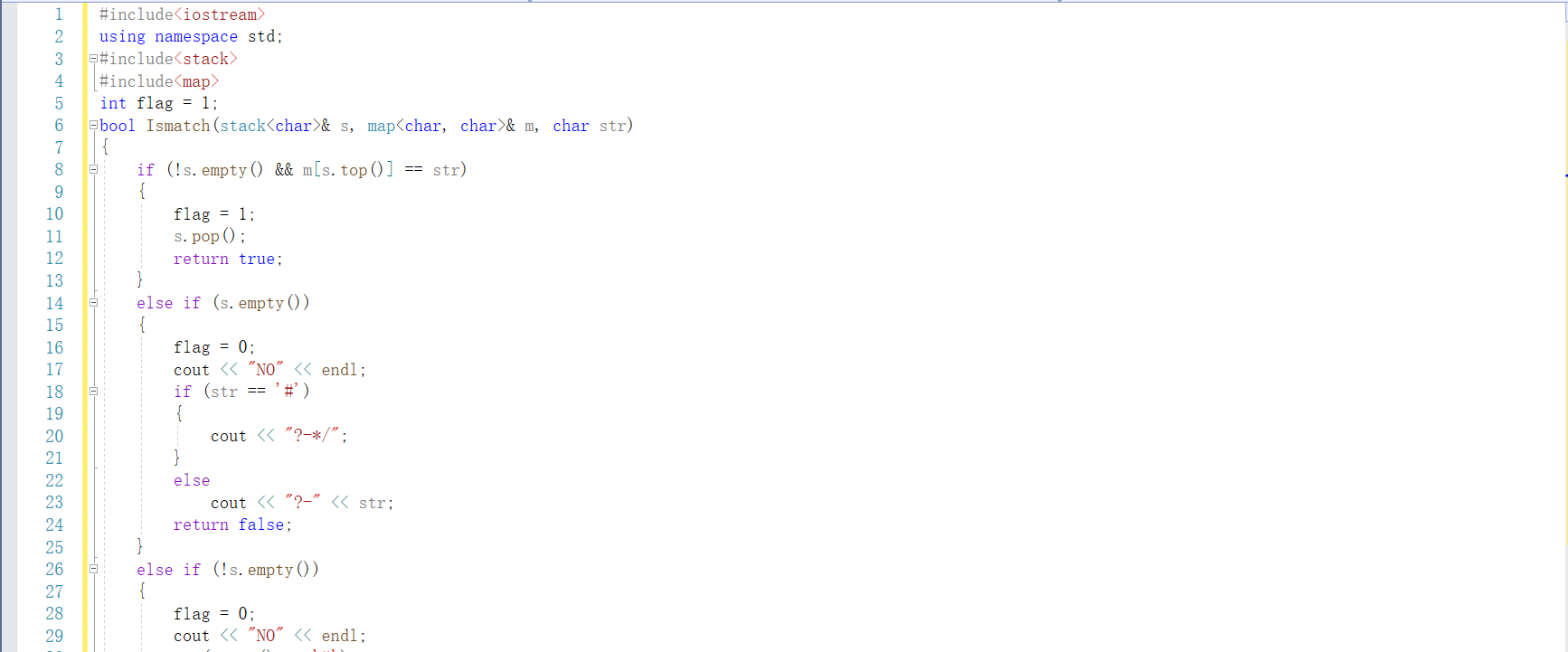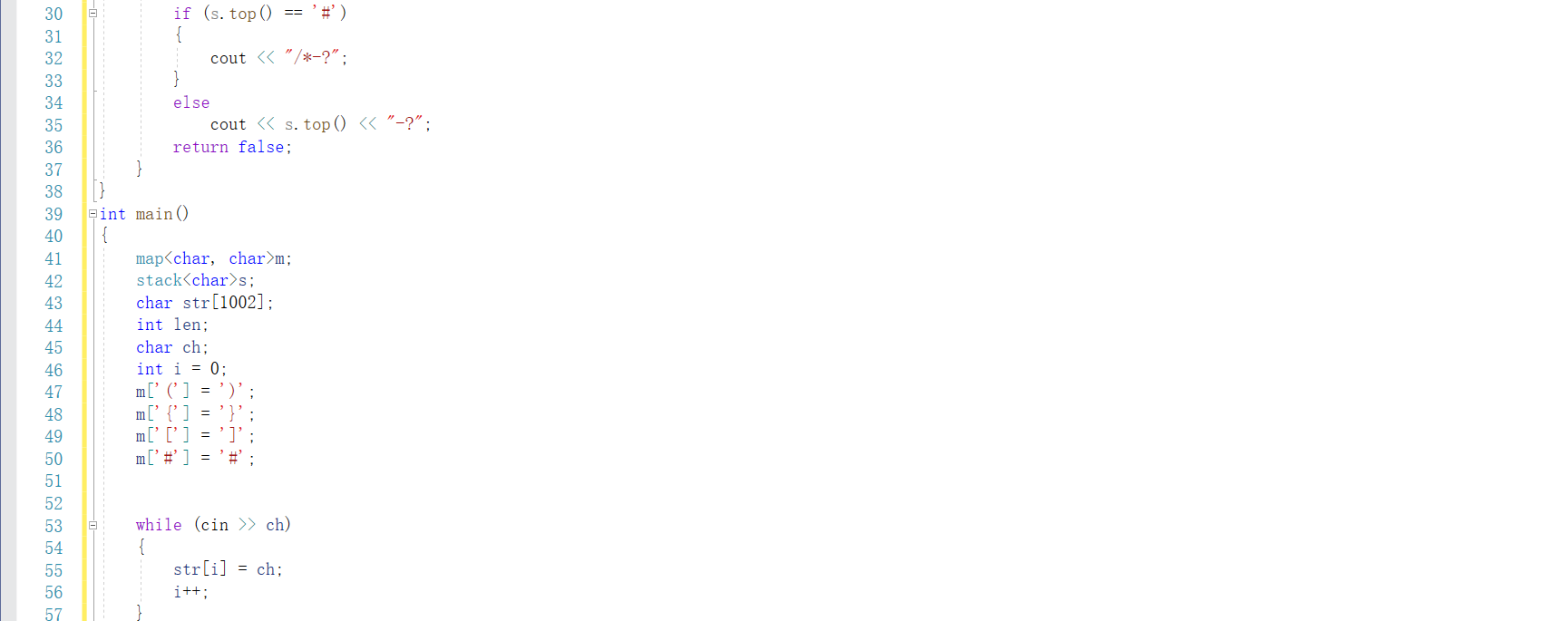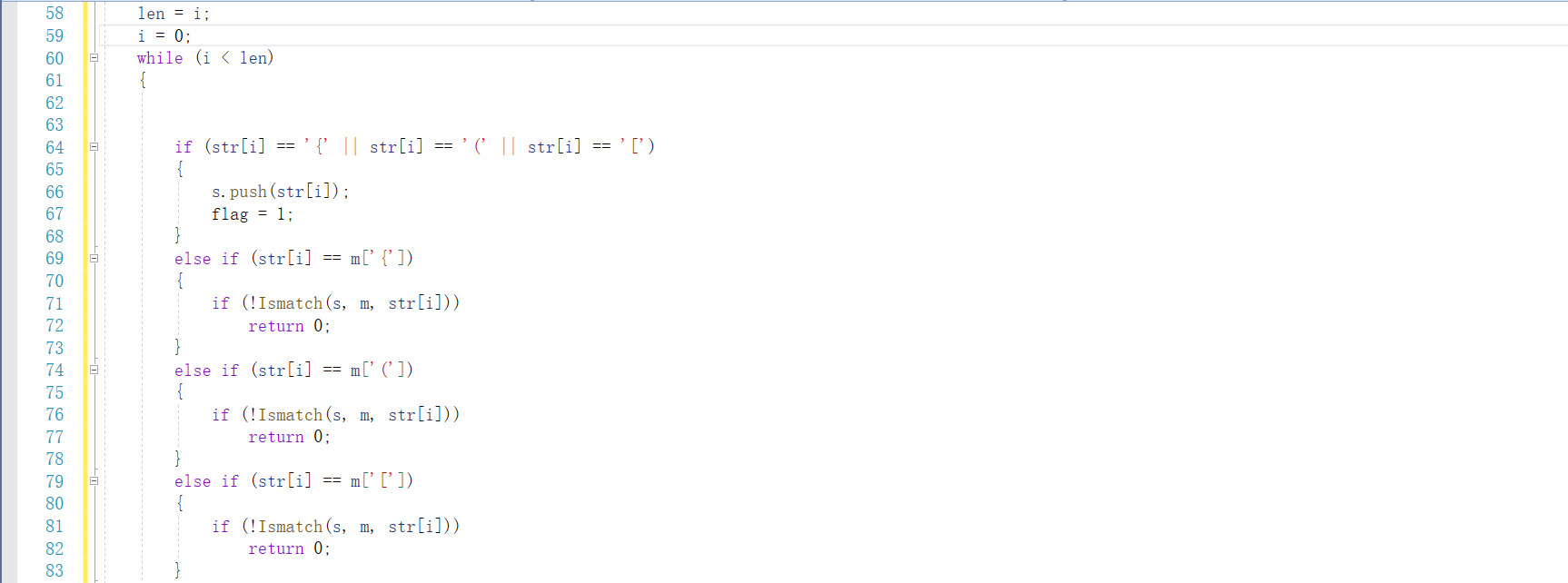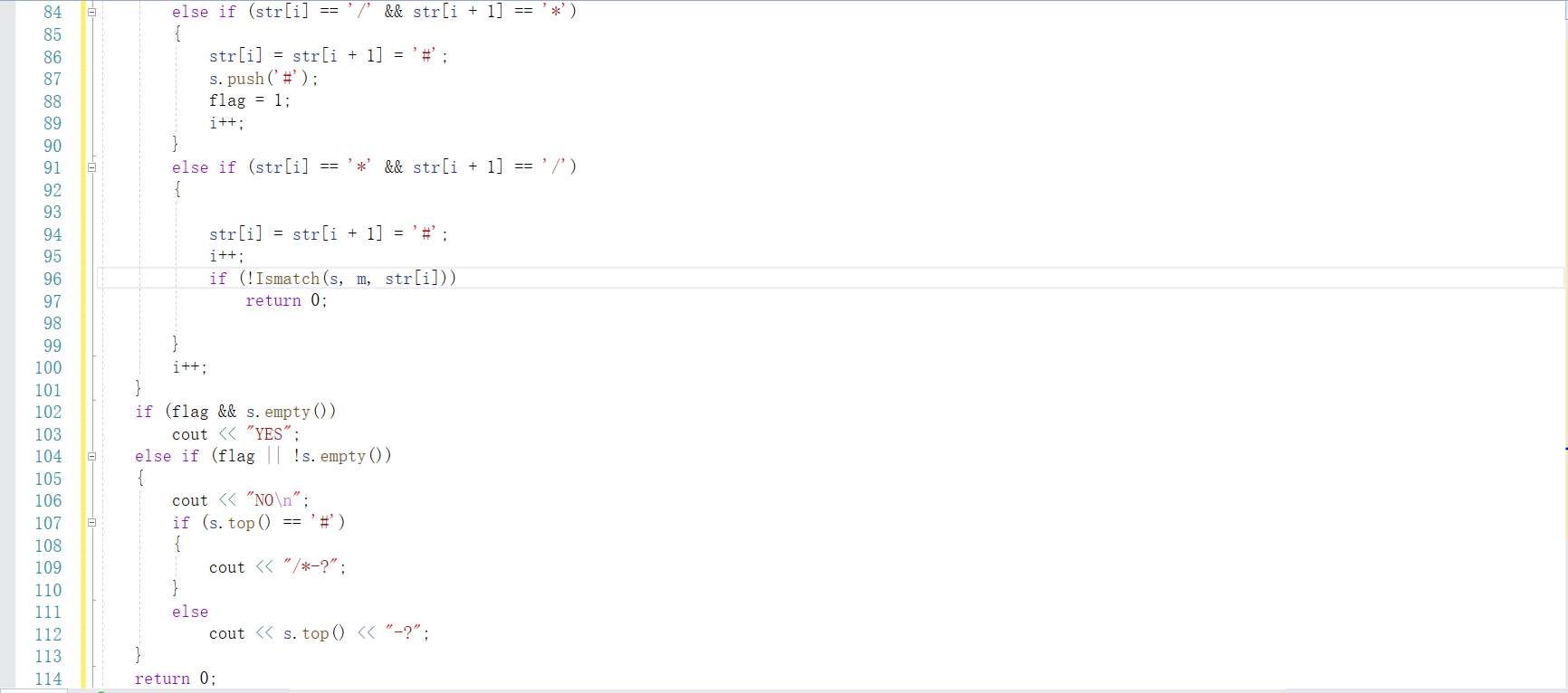### 2.1.2 PTA提交列表及说明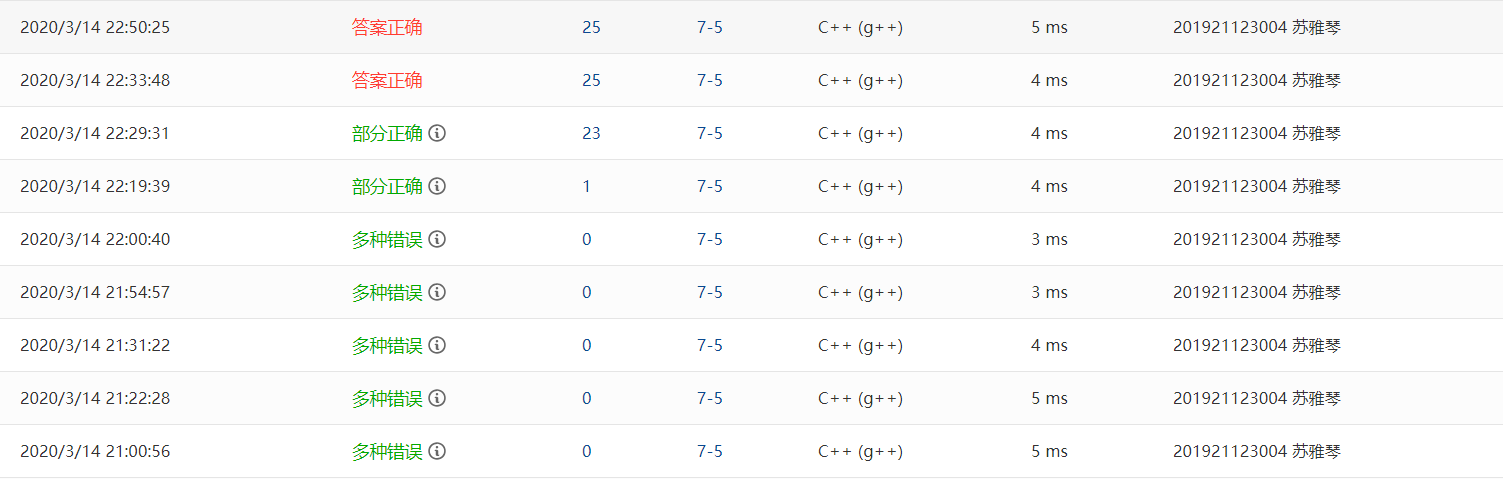• 多种错误：一开始，以为这道题和前面的括号匹配的代码差不多，就是多了/*，但是其实不一样的地方也很多，左括号和右括号的判断，导致输出的内容是不一样的，所以代码是错的。
• 然后写出来的，发现sample用vs测试是一样的，但是就是过不去，而且提示多种错误，挺方，一直想着左括号，右括号是不是有哪一种情况是被我漏掉的，但是这时候，格式错误，其实是对于连续数字的处理没有到位，并且忘记考虑小数点情况。
• 部分正确：最后一个坑嘛，就是对于符号的处理，就是正号本道题是不用输出的，但是负号是要输出的，而正负号在的位置只能是左括号的右边和数字的前面，所以加个判断就好了。

## 2.2题目：7-10 银行排队问题之单队列多窗口服务

### 2.2.1 代码截图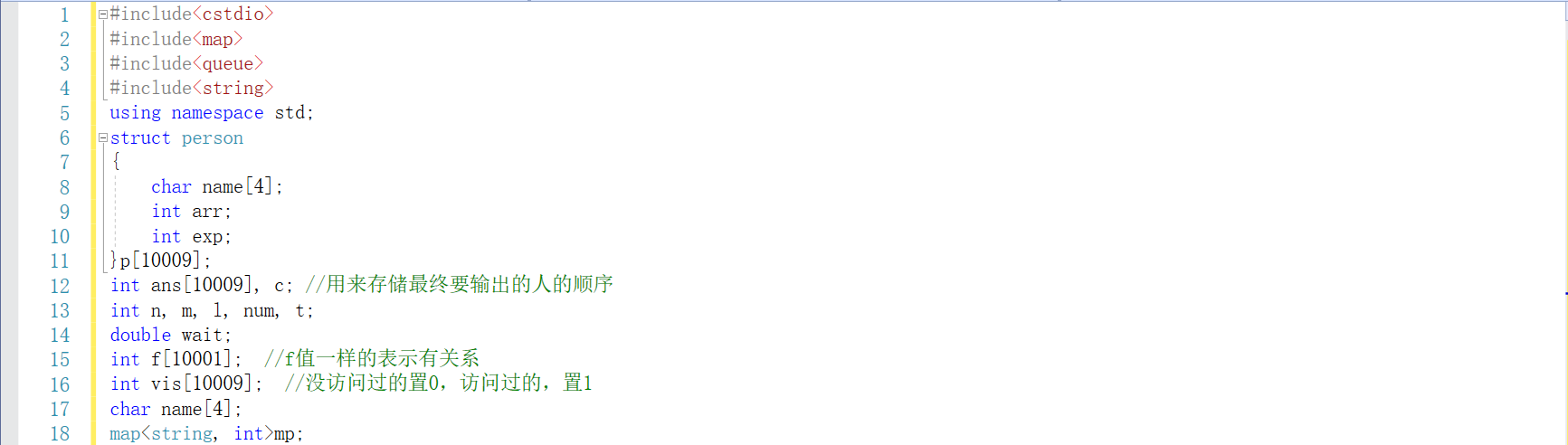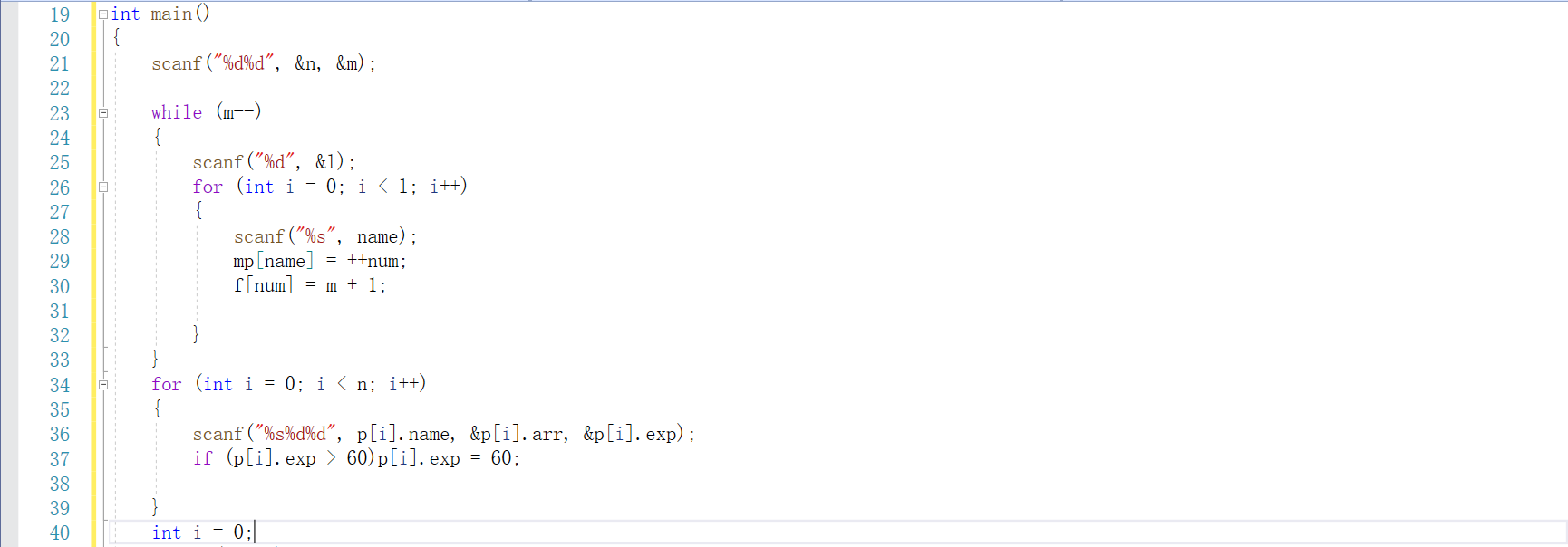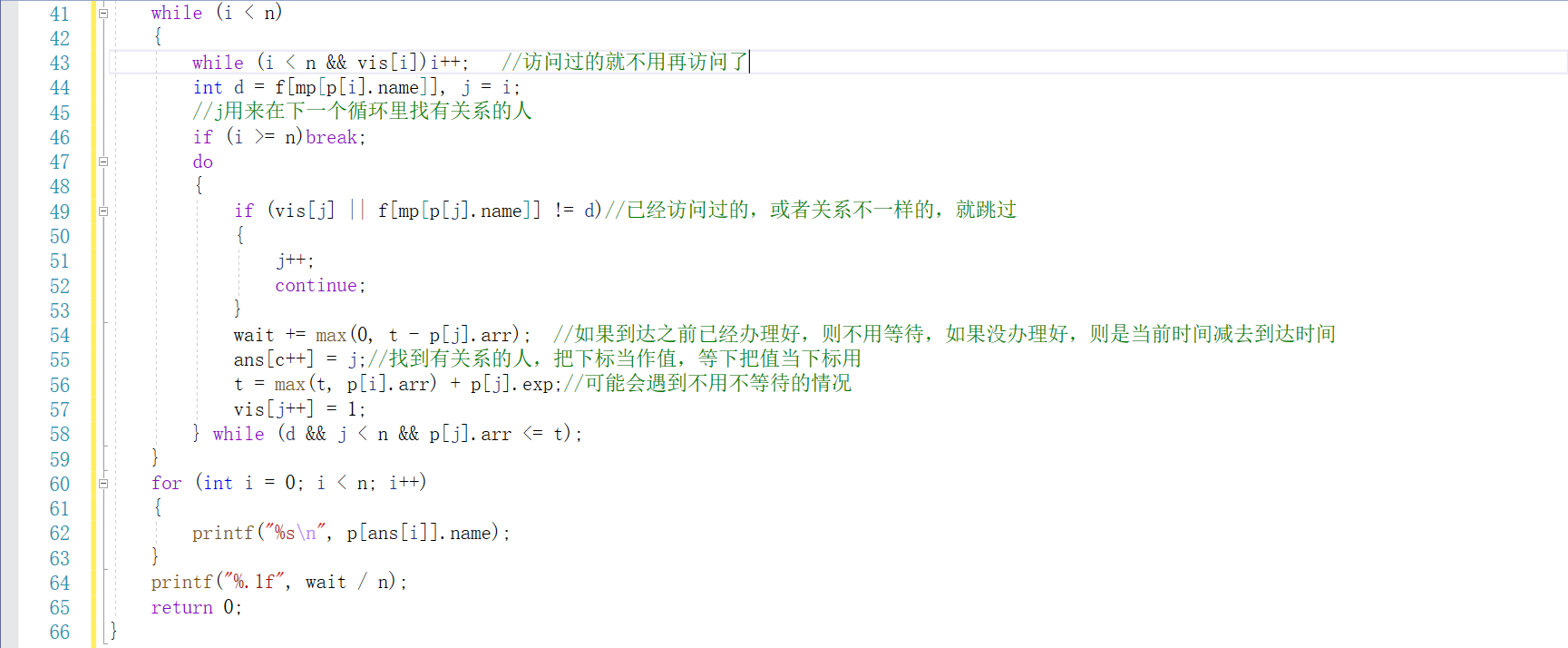### 2.1.2 PTA提交列表及说明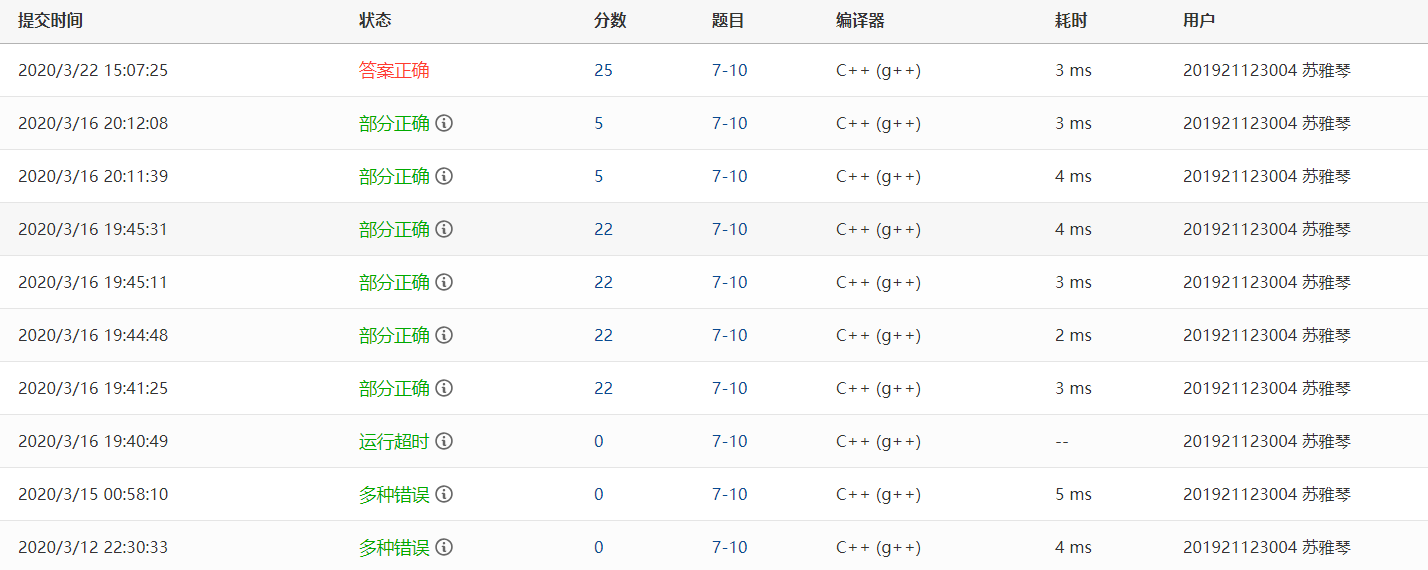• 多种错误：其实，觉得数学不好的人，做这种题，对我来说，好难，首先，由于有多个人和多个窗口，理清他们之间的对应关系以及关于时间的数学问题，就是挺难的一件事，（虽然是加减法，害），然后就是判断我该用什么样的数据结构去存储，我想了很久，于是打算用队列的vector容器
• 运行超时：其实用容器进行存储数据，然后进行调试的时候，我没有掌握到方法，当时以为用容器存储数据，是不能像数组那样，直接显示出每一项，但是后来偶然发现是可以的，但是当时不行，所以再调试的时候，很痛哭，就没有继续调试了。
• 做这道题，可能是我用的数据结构有问题，就是我存储的方式不太对，所以对于题目要求当有多个窗口可选择时，假设顾客总是选择编号最小的窗口,这个条件一直做不到，所以答案会错。

# 3.阅读代码

## 3.1 题目7-13 列车调度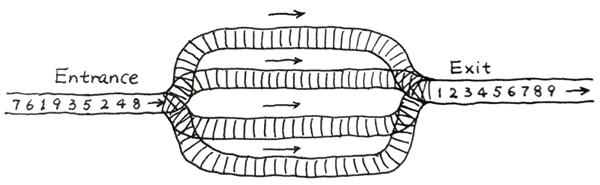• 输入格式：

​ 输入第一行给出一个整数N (2 ≤ N ≤105)，下一行给出从1到N的整数序号的一 个重排列。数字间以空格分隔。

• 输出格式：

在一行中输出可以将输入的列车按序号递减的顺序调离所需要的最少的铁轨条数。

• 输入样例：

9
8 4 2 5 3 9 1 6 7


• 输出样例：
4


### 3.1.0 代码截图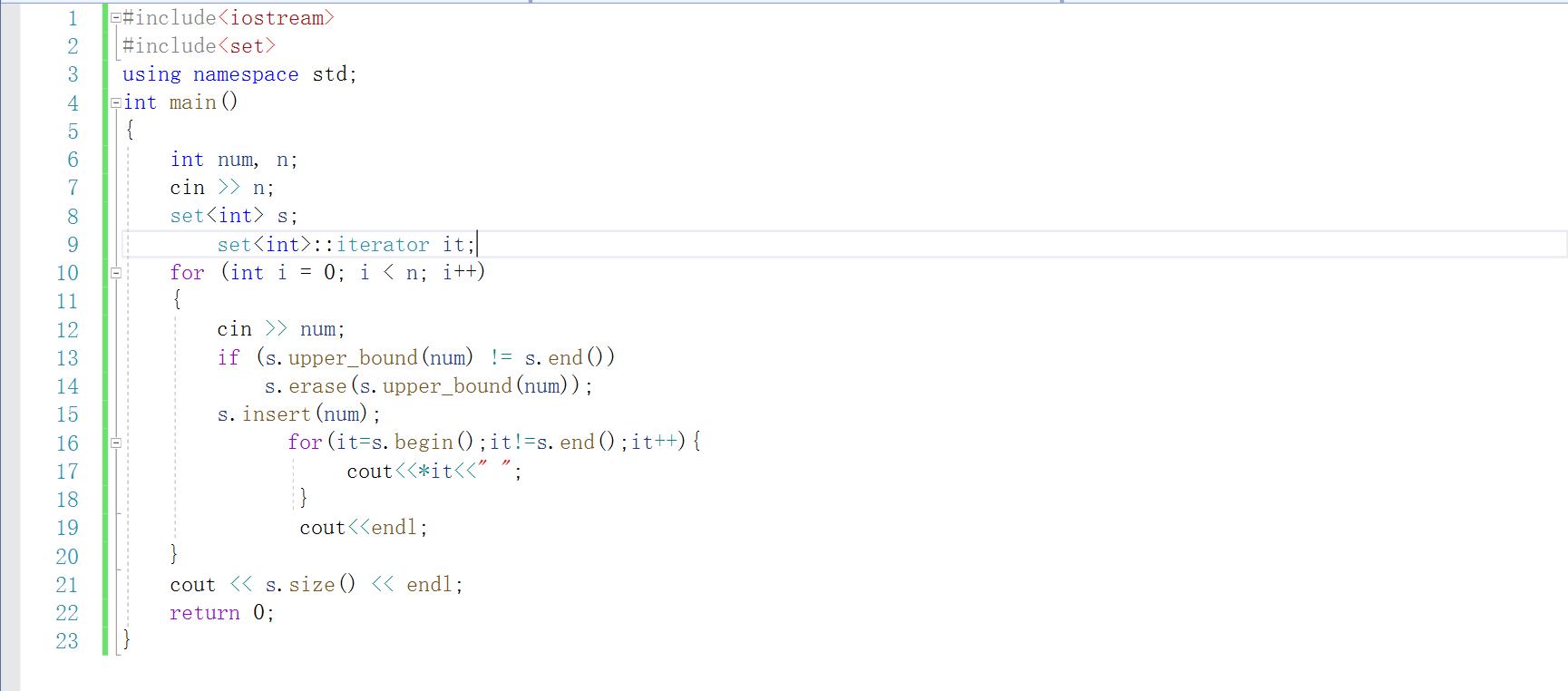### 3.1.1 设计思路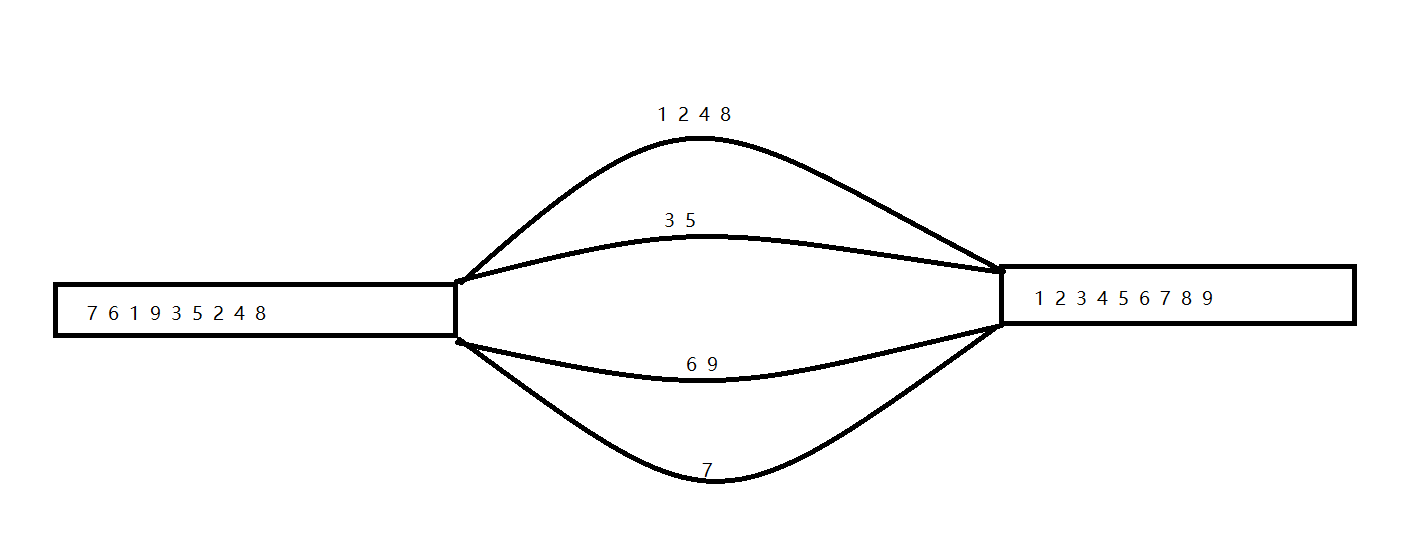### 3.1.2伪代码

定义set容器s

cin>>n;
for  i=0 to i<n
输入数字num
if(查找第一个大于或等于num的数)
找到则删除这个数
end if
将num插入到set容器中
end  for



### 3.1.3 运行结果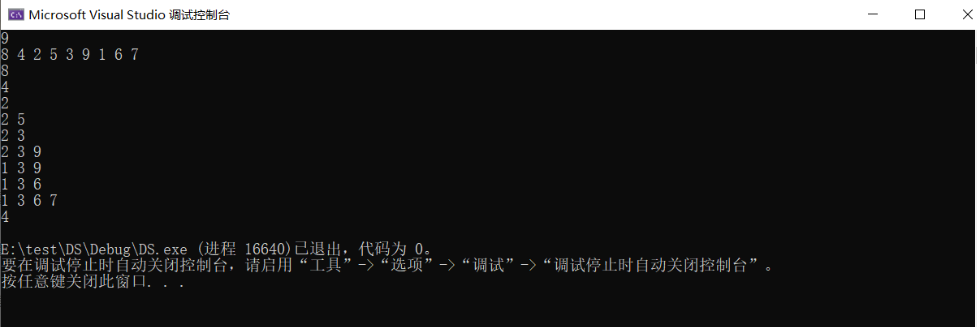## 3.2 题目 Bit Compressor

• 题目：

• 数据压缩的目的是为了减少存储和交换数据时出现的冗余。这增加了有效数据的比重并提高了传输速率。有一种压缩二进制串的方法是这样的：
将连续的n个1替换为n的二进制表示（注：替换发生当且仅当这种替换减少了二进制串的总长度）
（译者注：连续的n个1的左右必须是0或者是串的开头、结尾）
比如：11111111001001111111111111110011会被压缩成10000010011110011。原串长为32,被压缩后串长为17.
这种方法的弊端在于，有时候解压缩算法会得到不止一个可能的原串，使得我们无法确定原串究竟是什么。请你写一个程序来判定我们能否利用压缩后的信息来确定原串。给出原串长L，原串中1的个数N，以及压缩后的串。
L<=16 Kbytes，压缩后的串长度<=40 bits。

• 输入格式

第一行两个整数L,N，含义同问题描述
第二行一个二进制串，表示压缩后的串

• 输出格式

输出"YES"或"NO"或"NOT UNIQUE"（不包含引号）
分别表示：
YES：原串唯一
NO：原串不存在
NOT UNIQUE：原串存在但不唯一

样例1：
32 26
10000010011110011

9 7
1010101

14 14
111111


样例1：YES



### 3.2.0代码截图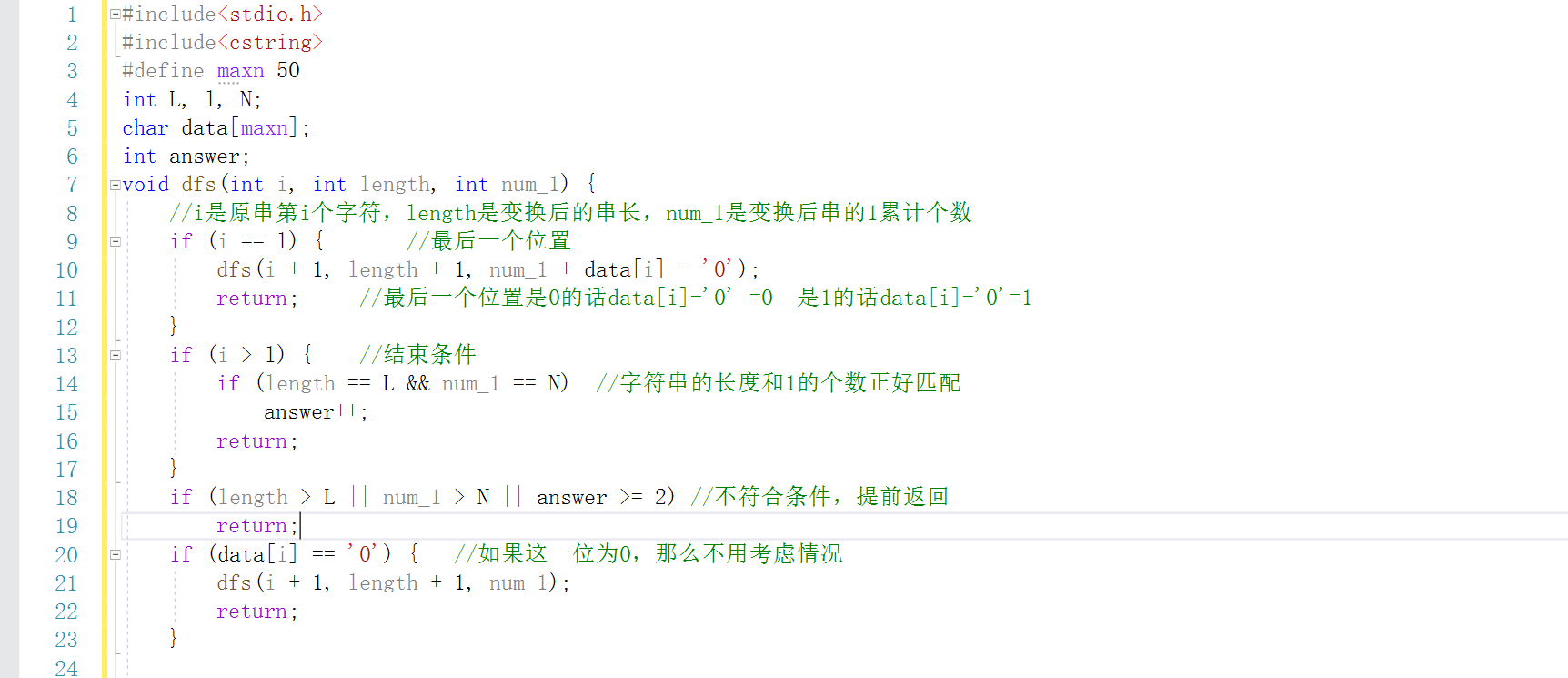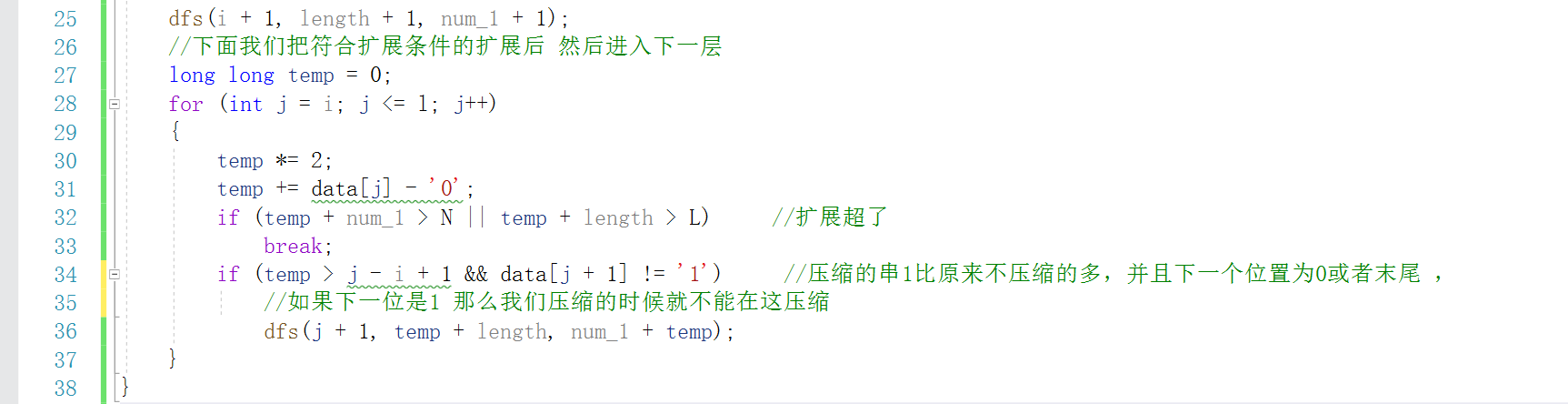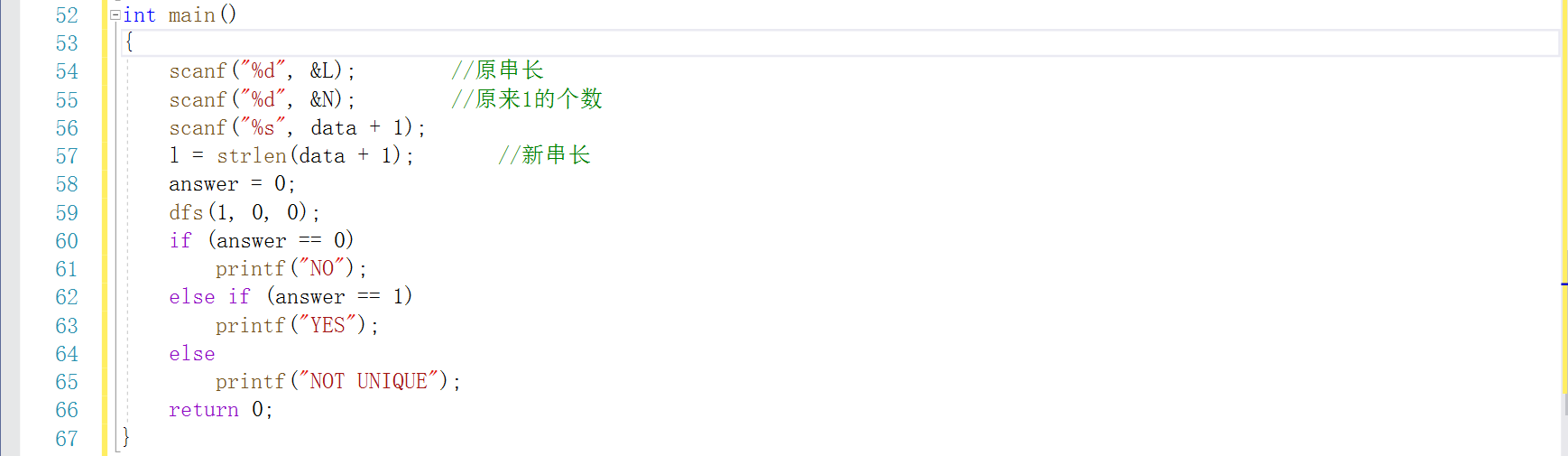### 3.2.2 伪代码

int main()
{
输入原创长和原创长中原来1的个数
输入压缩后的字符串

进入df函数
dfs(1,0,0);
}
i是原创第i个字符，length是变换后的串长，也就是我要求的原长长度，（不一定和输入的原长相等）num_1就是1的个数
void dfs(int i,int length,int num_1)
{
先正向进行搜索

if(如果遍历到刚好最后一个位置)
dfs(i+1,lenght+1,num_1+data[i]);
return ;
end if

if(有可能遍历的位置比压缩后的最大长度大一)
if(判断此时我们求出来的串长和1的个数是否与输入一样)
end if
return;
end if

然后是几个if语句，用来判断不用压缩的位置和可能压缩的位置

然后前面的代码是用于判断可能压缩的位置

下面的代码就是对于压缩的字符串中，从后面找到每一个1，进行回溯再深度搜索，算出原长

temp=0；用来保存压缩前1的个数

for int j=i to j<=l
temp*=2；
temp+=data[j]-'0';
//二进制转十进制，就是压缩前1的个数

if(解压缩的编码长度或1的个数大于原来的，不可行)
break;
end if
if(压缩的串1比原来的多，并且下一个位置为或末尾，就继续深搜)
dfs(j+1,temp+length,num_1+temp);

}


### 3.2.3运行结果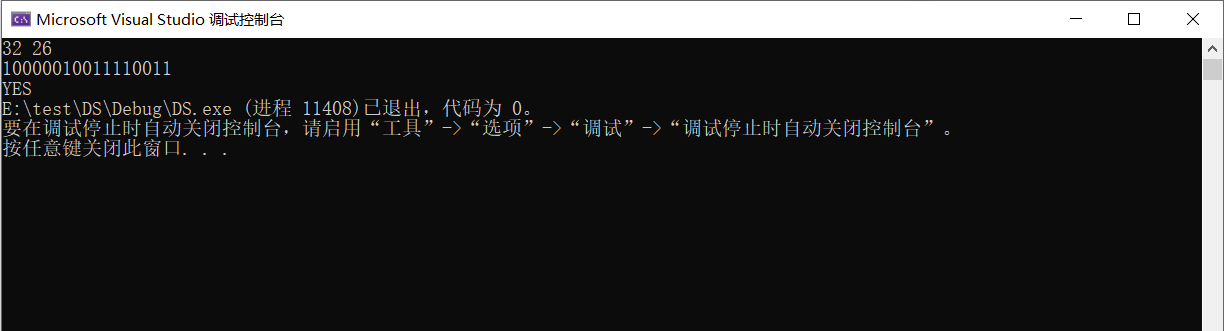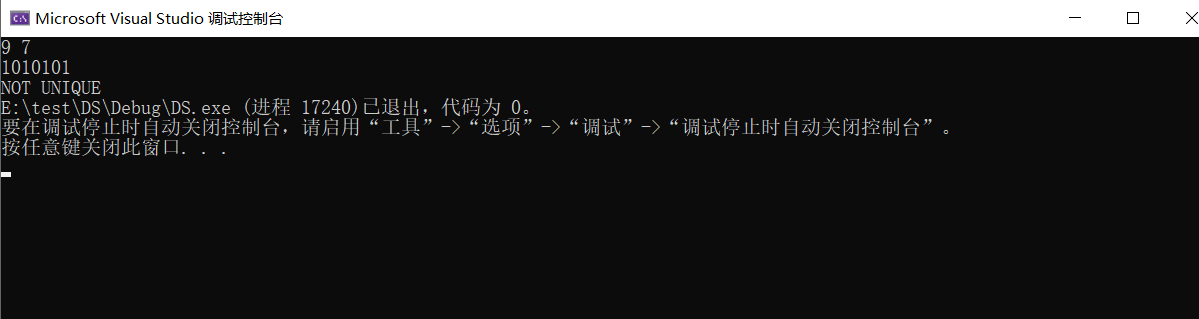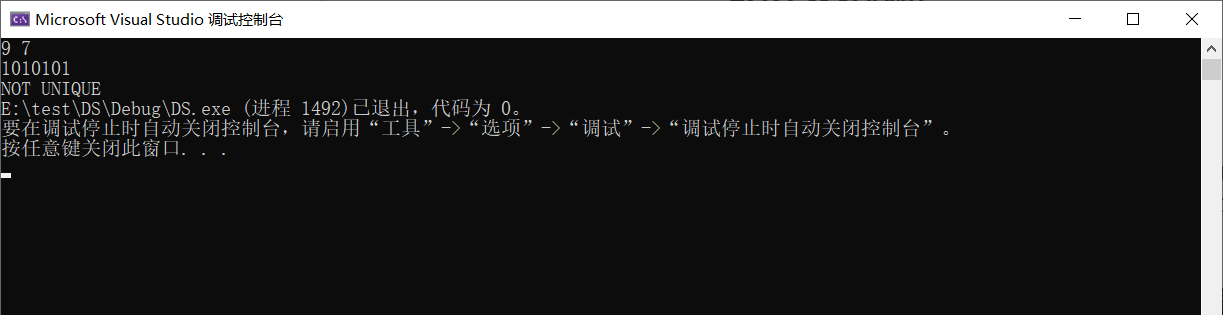### 3.2.4 分析该题目解题优势及难点

• 这个代码是剪枝问题，我上一个博客写的也是这个内容，不过感觉两个东西的风格完全不一样，这道题其实很好的利用了递归函数，说明写这个的人，，递归超级厉害，利用递归进行回溯，然后再深度搜索，调试的时候其实觉得很烧脑的一个东西，并且，这道题要先知道说，什么样的情况，我们是不需要压缩的，什么样的情况，我们要压缩，在回溯的时候，也要判断结束条件等等，所以利用递归，真的对一个人的能力要求很大。害，大佬的世界，不配拥有。

• ps：这道题，其实是我个人的看法，准不准确，我也不知道。

posted @ 2020-03-22 19:43  是啾咪呀。  阅读(380)  评论(0编辑  收藏  举报